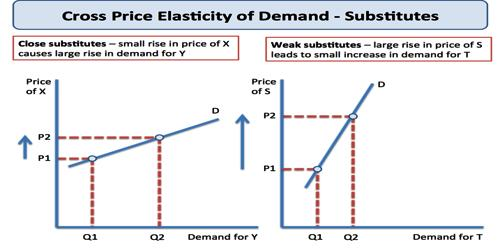Economics

# Concept of Cross Elasticity of DemandConcept of Cross Elasticity of Demand

Cross elasticity of demand is the relation between the percentage change in demand for a commodity to the percentage change in the price of related commodity. It is an economic concept that measures the responsiveness in the quantity demand of one good when a change in price takes place in another good. The cross elasticity of demand between good A and B is:

Cross elasticity = % change in quantity demanded for A good / % change to price of B good.

Assume products A and B are complements, meaning that an increase in the demand for A accompanies a decrease in the quantity demanded for B. Therefore, if the price of product B decreases, the demand curve for product A shifts to the right reflecting an increase in A’s demand, resulting in a negative value for the cross elasticity of demand. The exact opposite reasoning holds for substitutes.Types of Cross Elasticity of Demand

Theoretically, there are two types of cross elasticity of demand:

1. Positive Cross Elasticity Of Demand

In the case of substitute goods, the cross elasticity of demand is positive. If the price of tea rises, it will lead to increase in the demand for coffee. Similarly, a fall in price of tea will cause a decrease in the demand for coffee.

When goods are substitute of each other then cross elasticity of demand is positive. In other words, when an increase in the price of Y leads to an increase in the demand of X. For instance, with the increase in price of tea, demand of coffee will increase.

1. Negative Cross Elasticity Of Demand

In the case of complementary goods, cross elasticity of demand is negative. If the price of car rises, it will lead to decrease in the demand for petrol. Similarly, the fall in the price of car will bring the increase in the demand for petrol. Since, the price and demand change in opposite direction, the cross elasticity of demand is negative.

In case of complementary goods, cross elasticity of demand is negative. A proportionate increase in price of one commodity leads to a proportionate fall in the demand of another commodity because both are demanded jointly.

1. Zero:

Cross elasticity of demand is zero when two goods are not related to each other. For instance, increase in price of car does not affect the demand of cloth. Thus, cross elasticity of demand is zero.

Information Source: